# C++Splay实现过程（附代码）

Tips:本文的Splay不含维护区间的方法，以后会另开文章介绍

_____________________________________________________________________________________________________

Splay，又称伸展树，是平衡树的一种（蒟蒻我也只会写一种QWQ）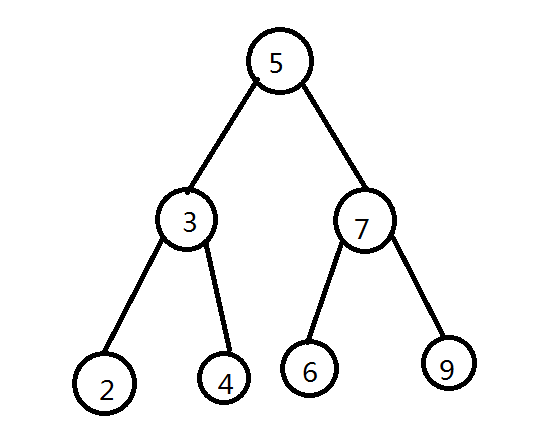（画图随便画的，很丑QWQ）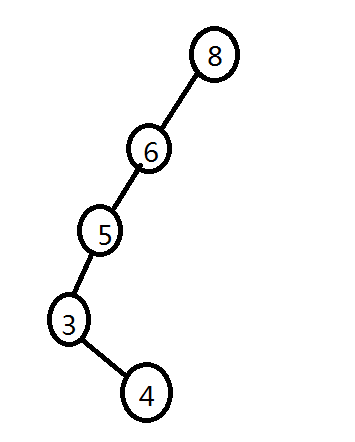_____________________________________________________________________________________________________

int son[x][0/1];-->节点x的左儿子(0)和右儿子(1)
int val[x];- ->节点x的值
int cnt[x];-->有多少个这个值
int size[x]; -->节点x所在子树元素个数
int fa[x]; -->节点x的父亲节点的下标

之后再确认些基本操作

1.

1 bool Dir(int x){ return son[fa[x]]==x; }

1 bool Dir(int x){ return val[x]>val[fa[x]]; }

Tips：由于bool类型的性质，当返回的逻辑式是正确的就会返回1，否则就会返回0

2.

void pushup(int x){ size[x]=size[son[x]]+size[son[x]]+cnt[x]; }

_____________________________________________________________________________________________________

1.当前节点的爷爷节点，即父节点的父节点，原来指向父节点的连边现在指向了当前节点，毕竟两点位置交换了；

2.当前节点的儿子变成了父节点，那么父节点应该插在左边还是右边呢？运用刚才上面所说的Dir操作，得出当前节点在父节点的位置情况，也就是大小关系，反过来就可以了，比如当前节点原来是父节点的左儿子，那旋转后父节点一定会成为自己的右儿子，因为右儿子一定是比自己大的嘛，而自己原来是左儿子说明父节点比自己大。

3.当前节点的儿子由于要变成父节点，原来这个位置的子节点就要变成父节点的子节点，插入的位置与当前节点原来在父节点的位置是相同的。

1 void rotate(int x){
2
3     int y=fa[x],z=fa[y],k=Dir(x),w=son[x][k^1];
4     son[z][Dir(y)]=x; fa[x]=z;
5     son[x][k^1]=y; fa[y]=x;
6     son[y][k]=w; fa[w]=y;
7     pushup(x); pushup(y);
8
9 }

_____________________________________________________________________________________________________

Splay就是通过这一操作不断在每次操作后将操作的节点伸展至根从而来维护这条路径上的平衡（不理解可以自己列个图），由于每次都这样保证了整棵树的平衡，伸展操作一般都只会花费O(log n)的时间。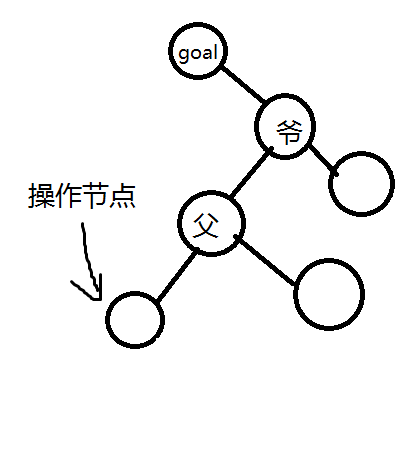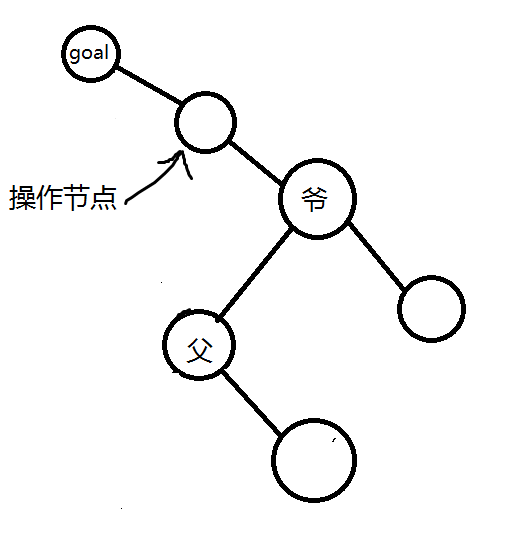1 inline void splay(int x,int goal=0){//没有填写伸展目标是默认为根节点
2
3     while (fa[x]!=goal){
4         int y=fa[x],z=fa[y];
5         if (z!=goal) {
6             if(Dir(x)==Dir(y)) rotate(y);
7             else rotate(x);
8         }
9         rotate(x);
10     }
11     if(!goal) Root=x;//当前节点伸展目标为根，更新根节点位置
12 }

_____________________________________________________________________________________________________

## 1.find（查询）操作

1 void find(int x){
2     if(!Root) return;
3     int cur=Root;
4     while(son[cur][x>val[cur]]&&val[cur]!=x)
5         cur=son[cur][x>val[cur]];
6     splay(cur);
7 }

## 2.insert（插入）操作

 1 void Insert(int x){
2     int cur=Root,p=0;
3     while(cur&&val[cur]!=x)
4     {
5         p=cur;
6         cur=son[cur][x>val[cur]];
7     }
8     if(cur) cnt[cur]++;
9     else {
10         cur=++tot;
11         if(p) son[p][x>val[p]]=cur;
12         son[cur]=son[cur]=0;
13         val[cur]=x;fa[cur]=p;
14         cnt[cur]=size[cur]=1;
15     }
16     splay(cur);
17 } 

## 3.求x的前驱/后驱操作

 1 inline int Last(int x)//求前驱 (前驱定义为小于x，且最大的数)
2 {
3     find(x);
4     if(val[Root]<x) return Root;//x不存在，根就可能为前驱/后驱
5     int cur=son[Root];
6     while(son[cur]) cur=son[cur];
7     splay(cur);
8     return cur;
9
10 }
11
12 inline int Next(int x)//求后驱 (后继定义为大于x，且最小的数)
13 {
14     find(x);
15     if(val[Root]>x) return Root;
16     int cur=son[Root];
17     while(son[cur]) cur=son[cur];
18     splay(cur);
19     return cur;
20 }
21  

## 4.Delete（删除）操作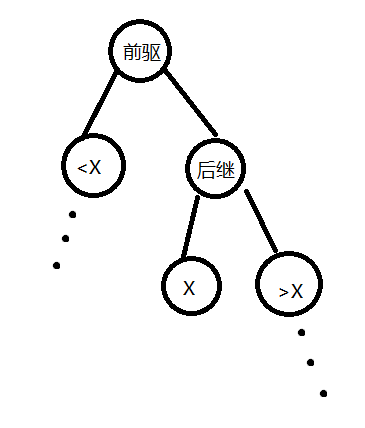1 void Delete(int x){
2     int L=Last(x),R=Next(x);
3     splay(L);splay(R,L);
4     int del=son[R];
5     if(cnt[del]>1) <% cnt[del]--;splay(del); %>
6     else son[R]=0;
7     pushup(R);pushup(Root);
8 }

## 5.GetRank（查询排名）操作

1 int GetRank(int x)
2 {
3     find(x);
4     if(val[Root]<x) return size[son[Root]]+cnt[Root];//如果为前驱就把根节点的数量也算上
5     else return size[son[Root]];//此处和上面原本应该+1但是如上的原因-1所以抵消了
6 }

## 6.Kth（查询第K小）操作

 1 int Kth(int k){
2     int cur=Root;
3     while(1){
4         if(k<=size[son[cur]]&&son[cur])
5             cur=son[cur];
6         else
7             if(k>size[son[cur]]+cnt[cur]){
8                 k-=size[son[cur]]+cnt[cur];
9                 cur=son[cur];
10             }
11             else{
12                     splay(cur);
13                     return cur;
14                 }
15     }
16 }

_____________________________________________________________________________________________________

  1 #include<bits/stdc++.h>
2 using namespace std;
3 const int inf=6*200100;
4 int tot,Root;
5
6 int son[inf];// 0->left 1->right
7 int val[inf];// 节点的值
8 int cnt[inf];//有多少个这个值
9 int size[inf];// 所在子树元素个数
10 int fa[inf];// 父亲节点下标
11
12 inline bool Dir(int x){ return son[fa[x]]==x; }
13
14 inline void pushup(int x)<% size[x]=size[son[x]]+size[son[x]]+cnt[x]; %>
15
16 inline void rotate(int x){//旋转
17     int y=fa[x],z=fa[y],k=Dir(x),w=son[x][k^1];
18     son[y][k]=w; fa[w]=y;
19     son[z][Dir(y)]=x; fa[x]=z;
20     son[x][k^1]=y; fa[y]=x;
21     pushup(y);pushup(x);
22 }
23
24 inline void splay(int x,int goal=0){//没有填写伸展目标是默认为根节点
25
26     while (fa[x]!=goal){
27         int y=fa[x],z=fa[y];
28         if (z!=goal) {
29             if(Dir(x)==Dir(y)) rotate(y);
30             else rotate(x);
31         }
32         rotate(x);
33     }
34     if(!goal) Root=x;//当前节点伸展目标为根，更新根节点位置
35 }
36
37 void find(int x){//寻找x并将他伸展至根，如果x不存在，就会找到当前数的前驱或后继
38     int cur=Root;
39     while(son[cur][x>val[cur]]&&val[cur]!=x)
40         cur=son[cur][x>val[cur]];
41     splay(cur);
42 }
43
44 inline void Insert(int x){//插入
45     int cur=Root,p=0;
46     while(cur&&val[cur]!=x)
47     {
48         p=cur;
49         cur=son[cur][x>val[cur]];
50     }
51     if(cur) cnt[cur]++;
52     else {
53         cur=++tot;
54         if(p) son[p][x>val[p]]=cur;
55         son[cur]=son[cur]=0;
56         val[cur]=x;fa[cur]=p;
57         cnt[cur]=size[cur]=1;
58     }
59     splay(cur);
60 }
61
62 inline int Last(int x)//求前驱 (前驱定义为小于x，且最大的数)
63 {
64     find(x);
65     if(val[Root]<x) return Root;//x不存在，根就可能为前驱/后驱
66     int cur=son[Root];
67     while(son[cur]) cur=son[cur];
68     splay(cur);
69     return cur;
70
71 }
72
73 inline int Next(int x)//求后驱 (后继定义为大于x，且最小的数)
74 {
75     find(x);
76     if(val[Root]>x) return Root;
77     int cur=son[Root];
78     while(son[cur]) cur=son[cur];
79     splay(cur);
80     return cur;
81 }
82
83 void Delete(int x){
84     int L=Last(x),R=Next(x);
85     splay(L);splay(R,L);
86     int del=son[R];
87     if(cnt[del]>1) <% cnt[del]--;splay(del); %>
88     else son[R]=0;
89     pushup(R);pushup(Root);
90 }
91
92 int Kth(int k){
93     int cur=Root;
94     while(1){
95         if(k<=size[son[cur]]&&son[cur])
96             cur=son[cur];
97         else
98             if(k>size[son[cur]]+cnt[cur]){
99                 k-=size[son[cur]]+cnt[cur];
100                 cur=son[cur];
101             }
102             else{
103                     splay(cur);
104                     return cur;
105                 }
106     }
107 }
108
109 int GetRank(int x)
110 {
111     find(x);
112     if(val[Root]<x) return size[son[Root]]+cnt[Root];
113     else return size[son[Root]];
114 }
115 int main()
116 {
117     int N;
118     scanf("%d",&N);
119     Insert(INT_MAX);
120     Insert(-INT_MAX);
121     for(int i=1;i<=N;i++){
122         int opt,x;
123         scanf("%d%d",&opt,&x);
124         if(opt==1) Insert(x);
125         if(opt==2) Delete(x);
126         if(opt==3) <% find(x); printf("%d\n",GetRank(x));%>
127         if(opt==4) <% printf("%d\n",val[Kth(x+1)]);%>
128         if(opt==5) <% printf("%d\n",val[Last(x)]);%>
129         if(opt==6) <% printf("%d\n",val[Next(x)]);%>
130     }
131     return 0;
132 }
posted @ 2019-08-08 07:58  fluoce  阅读(584)  评论(0编辑  收藏  举报